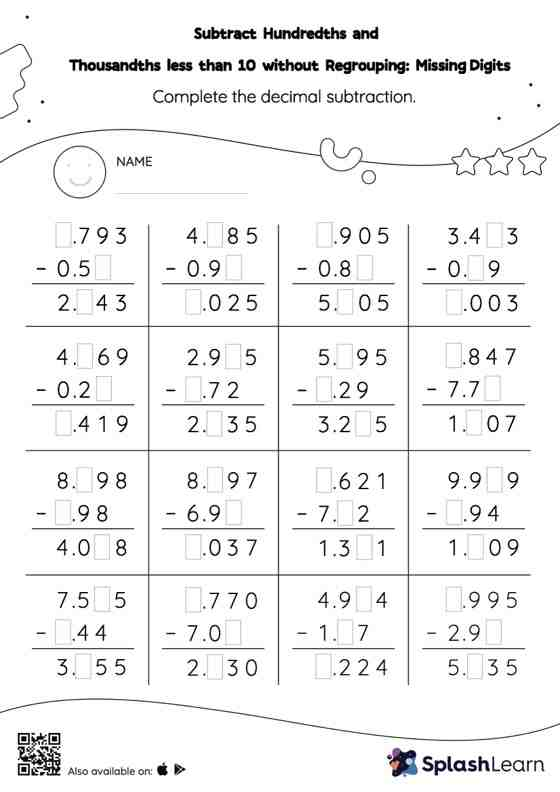# Subtract Hundredths and Thousandths less than 10 without Regrouping: Missing Digits Worksheet

Home > Subtract Hundredths and Thousandths less than 10 without Regrouping: Missing DigitsWhile subtracting decimals, students use the relationship between addition and subtraction to find the missing number. They do not need to regroup numbers in subtract hundredths and thousandths less than 10 without regrouping worksheet. As the worksheet uses the column method, it is helpful in getting students toward higher accuracy, especially with bigger numbers and in scenarios where regrouping is required.